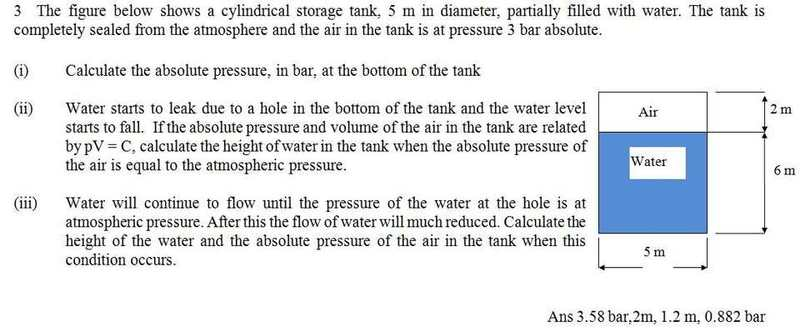# Pressure-Volume PV=C Air & Water in sealed vessel.

## Homework Statement## Homework Equations

I cannot work out the last part (iii) All other parts have been solved.

## The Attempt at a Solution

From part (ii) I found the new height of the water, and therefore the volume of water and air in the vessel. The Pressure of the water P1 cant be found using; rho.g.h+Patmos(for the water) and you know that P2 of the water is going to be Patmos.

Because you have 2 unknowns for the air, surely you must use V2=(P1V1/P2) However, this yields a greater volume that started with, indicating that the height has increase, not gone done, which is what does happen.

Bet its a really simple solution, but ive been on it for about 3 hours.

Cheers.

## Answers and Replies

Sunday Morning Bump.

Q_Goest
Science Advisor
Homework Helper
Gold Member
Hi Gorz. Yes, you have 2 unknowns for the air. But the pressure of the air is related to the height of the column of water. Note that the pressure at the bottom of the tank for iii is atmospheric pressure, so the air pressure is going to be less than atmospheric. Air pressure will be less than atm by rho*g*h. Note that water height also provides a function for the volume of air since the total volume of the tank is simply the summation of the water volume plus the air volume. Does that help?

Im still baffled by this. I understand the concept of the Air being a vacuum pressure and that you would find it using Patmos-rho.g.h, its getting the height that I cant do. Im not sure what you meen about the height providing a function for the volume.

Ive tried rearranging the V=phiD^2.h/4 and subbing it into the PV=C equation, but ive got nowhere with that.

Q_Goest
Science Advisor
Homework Helper
Gold Member
Hi Gorz. I don't have much time right now, but see if this helps. Rather than trying to derive the equation directly, sometimes it helps to do a calculation with actual values to see how you might go about determining the equation you need to derive.

Pick an actual value for H at random (let's say 1 meter) and using this value determine:
- The volume of air in the tank.
- The pressure of the air.
- The pressure at the bottom of the tank where the hole is.

Examine how you came up with the pressure of the air and water at the bottom and see if you can then go back and generalize the equations using variables. Hopefully once you do that, it will become obvious how to derive the equation you need.